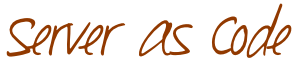# Add SSD as cache to ZFS on Linux

I just physically added a SSD into my home backup server and I would like to configure it as a ZFS l2arc cache device. Doing so will mean new files will be written to the SSD first, then the spinning disk later, and that recently used files will be accessed via the SSD drive instead of the slower spinning disks. Depending on your workload, this should make most disk operations faster.

In my case, the SSD is /dev/sdc.

``````root@storage:/home/curtis# lsblk /dev/sdc
NAME   MAJ:MIN RM   SIZE RO TYPE MOUNTPOINT
sdc      8:32   0 232.9G  0 disk
├─sdc1   8:33   0 232.9G  0 part
└─sdc9   8:41   0     8M  0 part
```
```

It’s a 240GB Samsung 840.

``````root@storage:/home/curtis# smartctl -a /dev/sdc
smartctl 6.2 2013-07-26 r3841 [x86_64-linux-3.13.0-24-generic] (local build)
Copyright (C) 2002-13, Bruce Allen, Christian Franke, www.smartmontools.org

=== START OF INFORMATION SECTION ===
Model Family:     Samsung based SSDs
Device Model:     Samsung SSD 840 Series
Serial Number:    S14GNEACC11801K
LU WWN Device Id: 5 002538 5500d4588
Firmware Version: DXT06B0Q
User Capacity:    250,059,350,016 bytes [250 GB]
Sector Size:      512 bytes logical/physical
Rotation Rate:    Solid State Device
Device is:        In smartctl database [for details use: -P show]
ATA Version is:   ACS-2, ATA8-ACS T13/1699-D revision 4c
SATA Version is:  SATA 3.1, 6.0 Gb/s (current: 3.0 Gb/s)
Local Time is:    Wed Jul  2 18:57:51 2014 MDT
SMART support is: Available - device has SMART capability.
SMART support is: Disabled

SMART Disabled. Use option -s with argument 'on' to enable it.
(override with '-T permissive' option)
```
```

Now let’s simply add it to the tank zpool. Note that “-f” means force, as this SSD was previously used with other file systems.

``````root@storage:/home/curtis# zpool add -f tank cache sdc
```
```

Hasn’t even been used!

``````root@storage:/home/curtis# zpool iostat -v tank
capacity     operations    bandwidth
----------  -----  -----  -----  -----  -----  -----
tank         757G   635G      0      0    262  1.25K
mirror     757G   635G      0      0    262  1.25K
sdb         -      -      0      0  1.35K  1.77K
sdd         -      -      0      0  1.82K  1.77K
cache           -      -      -      -      -      -
sdc        400K   233G      0      0    595  1.76K
----------  -----  -----  -----  -----
```
```

Fio is my favorite disk performance tool, so lets use that to test the new cache device. Note that this is just a basic, example fio test. Interestingly ZFS doesn’t support “direct=1”.

``````root@storage:/home/curtis# cat random-rw.fio
[random_rw]
rw=randrw
size=1024m
directory=/tank/bup
root@storage:/home/curtis# fio random-rw.fio
random_rw: (g=0): rw=randrw, bs=4K-4K/4K-4K/4K-4K, ioengine=sync, iodepth=1
fio-2.1.3
Starting 1 process
random_rw: Laying out IO file(s) (1 file(s) / 1024MB)
Jobs: 1 (f=1): [m] [99.1% done] [10468KB/10444KB/0KB /s] [2617/2611/0 iops] [eta 00m:01s]
random_rw: (groupid=0, jobs=1): err= 0: pid=1932: Wed Jul  2 18:43:00 2014
read : io=524704KB, bw=5017.9KB/s, iops=1254, runt=104567msec
clat (usec): min=3, max=3444, avg=16.18, stdev=34.60
lat (usec): min=3, max=3444, avg=16.42, stdev=34.61
clat percentiles (usec):
|  1.00th=[    5],  5.00th=[    7], 10.00th=[    8], 20.00th=[    9],
| 30.00th=[   10], 40.00th=[   11], 50.00th=[   12], 60.00th=[   13],
| 70.00th=[   14], 80.00th=[   15], 90.00th=[   36], 95.00th=[   41],
| 99.00th=[   53], 99.50th=[   63], 99.90th=[  189], 99.95th=[  462],
| 99.99th=[ 1848]
bw (KB  /s): min= 3200, max=17824, per=99.26%, avg=4979.99, stdev=1839.92
write: io=523872KB, bw=5009.1KB/s, iops=1252, runt=104567msec
clat (usec): min=9, max=5490, avg=772.32, stdev=244.37
lat (usec): min=9, max=5490, avg=772.62, stdev=244.38
clat percentiles (usec):
|  1.00th=[  213],  5.00th=[  318], 10.00th=[  414], 20.00th=[  620],
| 30.00th=[  692], 40.00th=[  748], 50.00th=[  804], 60.00th=[  852],
| 70.00th=[  916], 80.00th=[  972], 90.00th=[ 1020], 95.00th=[ 1048],
| 99.00th=[ 1112], 99.50th=[ 1144], 99.90th=[ 2928], 99.95th=[ 3152],
| 99.99th=[ 3440]
bw (KB  /s): min= 3408, max=17048, per=99.27%, avg=4972.41, stdev=1761.31
lat (usec) : 4=0.01%, 10=13.65%, 20=29.22%, 50=6.90%, 100=0.48%
lat (usec) : 250=0.93%, 500=5.35%, 750=14.01%, 1000=22.28%
lat (msec) : 2=7.04%, 4=0.12%, 10=0.01%
cpu          : usr=1.59%, sys=8.40%, ctx=132043, majf=0, minf=27
IO depths    : 1=100.0%, 2=0.0%, 4=0.0%, 8=0.0%, 16=0.0%, 32=0.0%, >=64=0.0%
submit    : 0=0.0%, 4=100.0%, 8=0.0%, 16=0.0%, 32=0.0%, 64=0.0%, >=64=0.0%
complete  : 0=0.0%, 4=100.0%, 8=0.0%, 16=0.0%, 32=0.0%, 64=0.0%, >=64=0.0%
issued    : total=r=131176/w=130968/d=0, short=r=0/w=0/d=0

Run status group 0 (all jobs):
READ: io=524704KB, aggrb=5017KB/s, minb=5017KB/s, maxb=5017KB/s, mint=104567msec, maxt=104567msec
WRITE: io=523872KB, aggrb=5009KB/s, minb=5009KB/s, maxb=5009KB/s, mint=104567msec, maxt=104567msec
```
```

Now the stats show some usage. The stats below are after a few test runs.

``````root@storage:/home/curtis# zpool iostat -v tank
capacity     operations    bandwidth
----------  -----  -----  -----  -----  -----  -----
tank         757G   635G      0     57  1.15K  6.68M
mirror     757G   635G      0     57  1.15K  6.68M
sdb         -      -      0     55  1.24K  6.68M
sdd         -      -      0     55  1.14K  6.68M
cache           -      -      -      -      -      -
sdc       11.7G   221G      0     54     79  6.81M
----------  -----  -----  -----  -----  -----  -----
```
```

That’s all it takes! Please let me know if you see anything incorrect.

Happy caching!